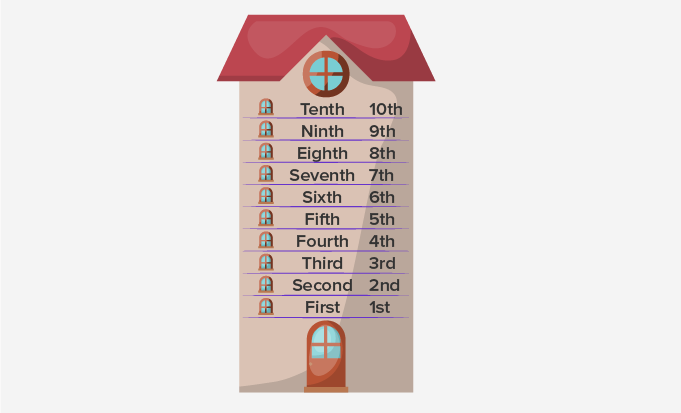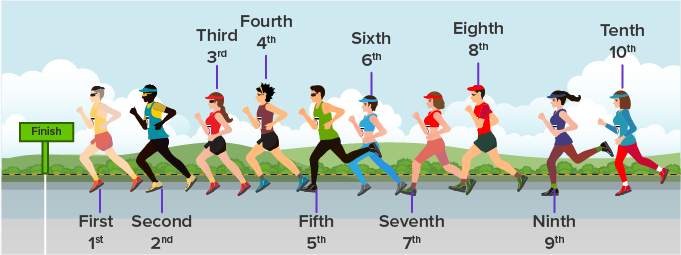Ordinal Numbers - Definition with Examples

The Complete K-5 Math Learning Program Built for Your Child

• 30 Million Kids

Loved by kids and parent worldwide

• 50,000 Schools

Trusted by teachers across schools

• Comprehensive Curriculum

Aligned to Common Core

Ordinal Numbers

Cardinal numbers

The numbers which give us the exact quantity of an object are called cardinal numbers. In other words, cardinal numbers answer “How many?”

For instance: The given picture shows 4 cars in a parking lot.  Hence, 4 or ‘four’ here is a cardinal number.Ordinal numbers

The numbers which give us the exact position of an object are called ordinal numbers. Ordinal numbers tell the position of an object rather than their quantity.

For instance:

Example 1- The given picture shows different floors in a building. We can use ordinal numbers to define their position.

The numbers 1st(First), 2nd(Second), 3rd(Third), 4th(Fourth), 5th(Fifth), 6th(Sixth), 7th(Seventh), 8th(Eighth), 9th(Ninth) and 10th(Tenth) tell the position of different floors in the building. Hence, all of them are ordinal numbers.Example 2-The given picture shows some athletes competing in a 400 m race. We can use ordinal numbers to define their position.The numbers 1st(First), 2nd(Second), 3rd(Third), 4th(Fourth), 5th(Fifth), 6th(Sixth), 7th(Seventh), 8th(Eighth), 9th(Ninth) and 10th(Tenth) tell the position of different athletes in the race. Hence, all of them are ordinal numbers.

Comparison Chart: Ordinal Numbers v/s Cardinal Numbers

 Cardinal Numbers Ordinal Numbers 1 One 1st First 2 Two 2nd Second 3 Three 3rd Third 4 Four 4th Fourth 5 Five 5th Fifth 6 Six 6th Sixth 7 Seven 7th Seventh 8 Eight 8th Eighth 9 Nine 9th Ninth 10 Ten 10th Tenth 11 Eleven 11th Eleventh 12 Twelve 12th Twelfth 13 Thirteen 13th Thirteenth 14 Fourteen 14th Fourteenth 15 Fifteen 15th Fifteenth

 Fun Fact- 11, 12 and 13 are the only numbers to use a suffix ‘–th’ but all other numbers ending with 1 use ‘–st’, 2 use ‘–nd’ and 3 use ‘–rd’.

Won Numerous Awards & Honors